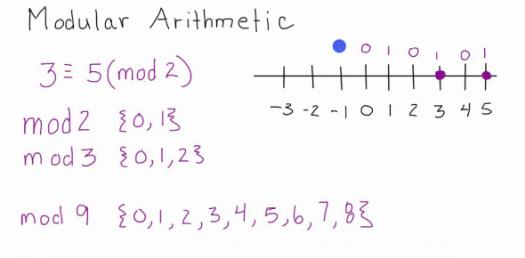# Arithmetic Practice Test! Trivia Quiz

20 Questions | Total Attempts: 64Settings.

Related Topics
• 1.
54 × 746 ÷ 32 = ?  (APPROXIMATE)
• A.

1259

• B.

1268

• C.

1196

• D.

1248

• E.

1236

• 2.
196 × 948 ÷ 158 = ?
• A.

1156

• B.

1200

• C.

1188

• D.

1176

• E.

None of these

• 3.
(786 × 64) ÷ 48 = ?
• A.

1050

• B.

1024

• C.

1048

• D.

1036

• E.

None of these

• 4.
3·5 + 11·25 × 4·5 – 32·5 = ?
• A.

18·275

• B.

21·625

• C.

32·375

• D.

25·45

• E.

None of these

• 5.
(2640 ÷ 48) × (2240 ÷ 35) = ?
• A.

3520

• B.

3515

• C.

3495

• D.

3490

• E.

None of these

• 6.
7414 + 3698 + 1257 + 1869 = ?
• A.

14328

• B.

14438

• C.

13428

• D.

13248

• E.

None of these

• 7.
(98360 + 25845 – 36540) ÷ 2500 = ?
• A.

36·585

• B.

30·082

• C.

32·085

• D.

35·066

• E.

None of these

• 8.
121, 117, 108, 92, 67, ?
• A.

31

• B.

29

• C.

41

• D.

37

• E.

None of these

• 9.
3, 23, 43, ?, 83, 103
• A.

33 33 33

• B.

53

• C.

63

• D.

73

• E.

None of these

• 10.
748, 737, 715, 682, 638, ?
• A.

594

• B.

572

• C.

581

• D.

563

• E.

None of these

• 11.
50, 26, 14, ?, 5, 3·5
• A.

6

• B.

8

• C.

10

• D.

12

• E.

None of these

• 12.
1, 9, 25, 49, ?, 169
• A.

100

• B.

64

• C.

81

• D.

121

• E.

None of these

• 13.
In how many different ways can the letters of the word ‘PRISE’ be arranged ?
• A.

90

• B.

60

• C.

180

• D.

120

• E.

None of these

• 14.
Sonali invests 15% of her monthly salary in insurance policies. She spends 55% of her monthly salary in shopping and on household expenses. She saves the remaining amount of Rs. 12,750. What is Sonali’s monthly income ? (A) (B) (C) (D) (E) Ans : (A)
• A.

Rs. 42,500

• B.

Rs. 38,800

• C.

Rs. 40,000

• D.

Rs. 35,500

• E.

None of these

• 15.
A milkman sells 120 litres of milk for Rs. 3360 and he sells 240 litres of milk for Rs. 6120. How much concession does the trader give per litre of milk, when he sells 240 litres of milk ?
• A.

Rs. 2

• B.

Rs. 3·5

• C.

Rs. 2·5

• D.

Rs. 1·5

• E.

None of these

• 16.
When 3626 is divided by the square of a number and the answer so obtained is multiplied by 32, the final answer obtained is 2368. What is the number ?
• A.

7

• B.

36

• C.

49

• D.

6

• E.

None of these

• 17.
The sum of the two digits of a two digit number is 14. The difference between the first digit and the second digit of the two digit number is 2. What is the product of the two digits of the two digit number ?
• A.

56

• B.

48

• C.

45

• D.

Cannot be determined

• E.

None of these

• 18.
A car runs at the speed of 50 kms per hour when not serviced and runs at 60 kms/hr. when serviced. After servicing the car covers a certain distance in 6 hours. How much time will the car take to cover the same distance when not serviced ?
• A.

8·2 hours

• B.

6·5 hours

• C.

8 hours

• D.

7·2 hours

• E.

None of these

• 19.
What approximate amount of compound interest can be obtaied on an amount of Rs. 9650 at the rate of 6 p.c.p.a. at the end of 3 years ?
• A.

Rs. 1737

• B.

Rs. 1920

• C.

Rs. 1720

• D.

Rs. 1860

• E.

Rs. 1843

• 20.
The number of employees in Companies A, B and C are in a rtio of 4 : 5 : 6 respectively. If the number of employees in the three Companies is increased by 25%, 30% and 50% respectively, what will be the new ratio of employees working in Companies A, B and C respectively ?
• A.

13 : 10 : 18

• B.

10 : 13 : 17

• C.

13 : 15 : 18

• D.

Cannot be determined

• E.

None of these